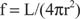## Apparent Magnitudes

Apparent magnitude (for which the symbol m is used) is a measure of how bright a star looks to the observer. In other words, it is a measure of a star's energy flux, the energy received per second per square meter at the position of the observer. The magnitude scale was created by Hipparchus, who grouped the stars he could see into six categories or magnitudes. Magnitude 1 stars are the brightest naked‐eye stars, magnitude 2 stars are fainter, magnitude 3 stars even fainter, and so forth, down to magnitude 6, the faintest stars visible to the naked‐eye. When it became possible to make reliable energy measurements of starlight, it was found that a difference of 1 magnitude corresponds to a ratio of brightnesses (or fluxes) of about 2.5 times. The modern magnitude scale makes this quantitatively very precise: By definition, a difference of 5 magnitudes corresponds to a brightness (flux) ratio of 100. In turn, a difference of 2.5 magnitudes corresponds to a brightness ratio of √100 = 10, and a difference of 1 magnitude corresponds to a brightness ratio of 5√100 = 2.512, and so forth. Magnitudes m therefore represent a logarithmic scale; for example, for two objects 1 and 2 with respective magnitude m 1 and m 2,where the brightness or flux (f) is measured in watts/m 2. Flux is related to both the intrinsic energy output of an object (its luminosity L) and the area (4πr 2) over which that energy has spread as it moves out to a distance r from the object:Therefore, an apparent magnitude depends on both the true luminosity and the distance of an object.

The original magnitude scale was limited to naked‐eye stars, but it can be applied to even brighter objects by extending to negative numbers (see Figure ). The apparent magnitude of the Sun is −26.8 and the full Moon –12.5. Fainter objects have larger positive numbers. The Hubble Space Telescope can detect objects as faint as m = +30 (24 magnitudes, or about four billion times fainter than the faintest naked‐eye stars).Figure 1

Apparent magnitude scale.

Originally, magnitudes were based on visible light; but with the use of filters, astronomers can measure brightnesses in specific parts of the spectrum. A U magnitude is one measured using the ultraviolet light of a star, B represents a blue magnitude, V a visual (yellow‐green part of the spectrum) magnitude, with R, I, J, K, M, and so on, representing ever longer wavelengths in which the measurement is made.